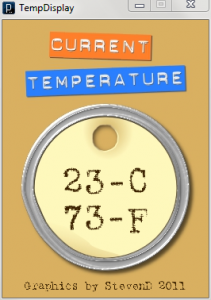## 2011年3月27日 星期日

### Processing 溫度顯示器晚上在 Hacker Highligts: Steven’s Temperature Display 這篇文章看到一個好玩的東西，Steven Dayton 根據 Jeremy Blum 做的 Arduino+I2C+Processing 溫度顯示器用 Processing 寫了一個很好看的溫度顯示器(如左圖)。

Steven Dayton 原版程式會顯示攝氏跟華氏溫度，我把它改成只顯示攝氏溫度，至於溼度就略過不管了。

#### 8 意見:

import processing.serial.*;
import cc.arduino.*;

Arduino arduino;
int ledPin = 13;

void setup()
{
//println(Arduino.list());
arduino = new Arduino(this, Arduino.list(), 57600);
arduino.pinMode(ledPin, Arduino.OUTPUT);
}

void draw()
{
arduino.digitalWrite(ledPin, Arduino.HIGH);
delay(1000);
arduino.digitalWrite(ledPin, Arduino.LOW);
delay(1000);
}

Processing訊息窗內出現的訊息如下:
Exception in thread "Animation Thread" java.lang.IllegalAccessError: tried to access class processing.core.PApplet\$RegisteredMethods from class cc.arduino.Arduino\$SerialProxy
at cc.arduino.Arduino\$SerialProxy.(Arduino.java:119)
at cc.arduino.Arduino.(Arduino.java:168)
at sketch_121006a.setup(sketch_121006a.java:34)
at processing.core.PApplet.handleDraw(PApplet.java:2103)
at processing.core.PGraphicsJava2D.requestDraw(PGraphicsJava2D.java:190)
at processing.core.PApplet.run(PApplet.java:2006)

import processing.serial.*;
import cc.arduino.*;
Arduino arduino;
int ledPin = 13;

void setup()
{
size(200,200);
//println(Arduino.list());
arduino = new Arduino(this, Arduino.list(), 57600);
arduino.pinMode(ledPin, Arduino.OUTPUT);
}

void draw()
{
fill(204, 153, 0);
ellipse(100,100,30,30);
arduino.digitalWrite(ledPin, Arduino.HIGH);
delay(1000);
fill(125);
ellipse(100,100,30,30);
arduino.digitalWrite(ledPin, Arduino.LOW);
delay(1000);
}

Cooper Maa 提到...

1. 就像 Blink without delay 的方法，利用 millis() 算經過的時間:

2. 試試這個網站包的 timer (功能還要加強，它沒有 repeat 功能，只有跑一次的功能)
http://www.learningprocessing.com/examples/chapter-10/example-10-5/

3. 利用 frameRate() 和 frameCount 變數

Error from inside the Android tools. check the console.

Cooper Maa 提到...

processing 程式可以直接轉成 android app, 但是我還是沒試出來 :(

Cooper Maa 提到...

processing 程式可以直接轉成 android app, 但是我還是沒試出來 :(